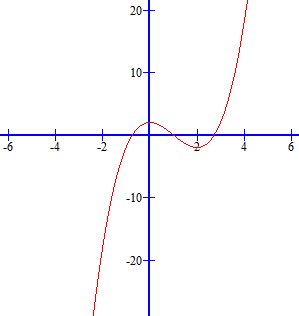### Sample Problem

a)If g(x)=x3-3x2+2, according to Descartes’s Rules of Signs, g(x) could have one positive real zero.

True

False

b)If g(x)=x3-3x2+2, according to Descartes’s Rules of Signs, g(x) could have one negative real zero.

True

False

#### Solution

Descartes’s Rule of Signs says that if f(x) is a polynomial with real coefficients and a constant term that is not zero, that the number of positive real zeros of f is either equal to the number

of variations in sign of f(x) or less than that number by an even integer.

g(x) has 2 variations in sign, therefore it can either have 2 positive real zeros or none (2 variations minus 2, an even integer)… but it cannot have one positive real zero.

g(-x)=(-x)3-3(-x)2+2=-x3-3x2+2

g(-x) has 1 variation in sign, therefore it has to have 1 negative real zero. (1 minus an even integer that is not 0, that is 2 gives a negative number… which is not positive).

If we check the graph of g(x),We can see it has 2 positive zeros and 1 negative zero, as Descartes’s Rule promised.